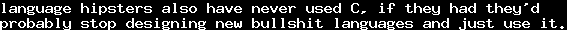# Adding superclasses to classes in Common LispWed, 02 Feb 2011 15:18:55 GMT

I wonder wheter any of the "modern" object systems and their reflection APIs can do this:

```;; define classes a and b
(defclass a () ())
(defclass b () ())

(defgeneric test (obj))
(defmethod test ((obj a)) 'a-method)
(defmethod test ((obj t)) 't-method)

;; the t-method is called, since b is not subclass of a
(assert (eql 't-method
(test (make-instance 'b))))

;; now we add the class a as an additional superclass
(reinitialize-instance
(find-class 'b)
:direct-superclasses (list (find-class 'a)))

;; and now, the a-method is called, since b is subclass of a
(assert (eql 'a-method
(test (make-instance 'b))))
```